## Indicators

•Cited by SciELO
•Access statistics

•Similars in SciELO

## Print version ISSN 1405-5546

### Comp. y Sist. vol.21 n.2 México Apr./Jun. 2017

#### http://dx.doi.org/10.13053/cys-21-2-2740

Articles

Bridging the Gap Between Model-Based Design and Reliable Implementation of Feedback-Based Biocircuits: A Systems Inverse Problem Approach

1 Control Department of CINVESTAV-IPN, Mexico City, Mexico

2 Instituto Politécnico Nacional, Centro de Investigación en Computación, Mexico City, Mexico

Abstract:

Our concern is the tuning of mathematical models describing rationally designed genetic biocir-cuits. Based on a deterministic lumped continuous-time approach, we propose a tuning methodology combining both exact algebraic parameter reconstruction and nonlinear parameter estimation of a given model supporting the design of a specific genetic biocircuit, i.e., we bridge the gap between model-based design and implementation as the solution of a systems inverse problem. As a proof of concept, our proposal is constrained to cyclic feedback systems characterizing synthesized transcriptional networks conditioned to display sustained oscillatory behavior. Our proposed methodology is illustrated via computer–based simu-lations involving the tuning of a state–based model describing a well–know cyclic feedback biocircuit: the celebrated repressilator. Tuning in our case is conceived as a procedure to adjust the parameter values of the mathematical model taking into account for this the actual behavior observed from the corresponding synthesized biocircuit.

Keywords: Systems biology; synthetic biology; tuning of mathematical models; algebraic parameter reconstruction; observer based system identification; synthetic transcriptional networks; cyclic feedback biocircuits

1 Motivation

This paper extends the discussion first presented in , following for this an approach focused on methodological issues. Our purpose is then to enlighten the limitations that are imposed by the unavoidable uncertainty on the value of the involved parameters, as far as synthetic biology designs are concerned.

The simple illustrative example that we use here in order to present our ideas leads us to conclude that no realistic synthetic biology design are possible at all, if not parameter estimation is included in the physical realization of the designed system. Synthetic biology asks then for hybrid designs, i.e., designs involving not only the biological designed system but also a computer-based parameter estimation module.

2 Introduction

Systems biology, the integrative systems–based contemporary post-genomic vision of biology, promotes the understanding of living organisms through the tinkerer’s approach, which is to say building life to understand it (and also to optimally profit from it), see for instance . Recent scientific and technological progress in molecular cell biology, genetic engineering, as well as in mathematical and computational modeling of biological systems and in biological engineering methodologies, offers the opportunity to rationally design and build genetic circuits as a way to understand existing biological functionalities, and even better: as a rational way to add new prescribed functionalities to existing biological organisms (see for instance [3, 19, 18, 9] and the references therein).

As is done in modern engineering, the current design approach followed by synthetic biology practitioners has mathematical modeling as the first step of the rational design procedure for the intended biocircuit (see for instance ). Going from a mathematical model to the realization of its corresponding physical system is a standard engi-neering approach ruling design on well–developed technological domains like electronic engineering, mechanical engineering, aerospace engineering, and computer engineering. It is then common to build highly consumed products (like cars or mobile phones) directly from computer-based design and computer-based simulation. However, as far as the physical realization of a mathematically modeled biocircuit is concerned, several factors challenge the approach followed by modern engineering. Inherent stochasticity of cellular bio–molecular systems, as well as many others factors (like technological limitations of the involved measurement processes), result in mismatch between the parameter values of the mathematically modeled system and the actual parameter values of the corresponding synthesized biological system. This mismatch moves the behavior of the synthesized system far away from the designed behavior coded by the guiding mathematical model, which reduces then the utility of mathematical modeling in biological engineering (particularly if the concerned model is constrained to be simple enough in order to be useful in terms of design and simulation).

Tuning of the mathematical model is an obvious tool when considering the minimization of the mismatch between the designed behavior and the displayed behavior. It is then necessary to reconstruct the actual parameter values of the designed system through measuring of the actual system’s behavior. In this paper we propose an approach based on exact algebraic parameter reconstruction combined with nonlinear algebraic parameter estimation to find the parameter values of cyclic feedback systems describing synthesized biocircuits. As the solution of an inverse problem, the tuning procedure finds the parameter values processing available information provided by the synthesized system. We conceive our methodology as a proof of concept and we constraint our exposition to the case of transcriptional networks conditioned to display measurement available for sustained oscillatory behavior, described in mathematical terms by cyclic feedback systems. We follow a deter-ministic lumped continuous–time approach, and we support our conclusions via computer-based simulations involving the well-known illustrative synthetic biocircuit: the repressilator.

The paper is organized as follows: Section 3 discusses the problem formulation. We briefly recall what is characteristic for the cyclic feedback nonlinear dynamical systems, and its application as a modeling tool for the description of a particular class of synthesized transcriptional networks. Moreover, we formulate the inverse problem in terms of the determination of a set of real parameters tuning a ordinary differential equations based mathematical model to force it to describe the sustained oscillatory behavior of the synthesized transcriptional network. As an illustration of our proposal we limit our exposition to the tuning of a mathematical model of the well–know synthetic cyclic feedback bio–oscillator known as the repressilator. We expose our tuning procedure in Section 4 and in Section 5 we illustrate it via computer– based based simulations involving the well–known synthetic biocircuit called the repressilator. We conclude the paper with some final remarks in Section 6.

3 Problem Formulation

In this section we recall the definition of cyclic feedback systems, and we restrict our exposition to the deterministic lumped continuous-time in-variant framework. Then, we discuss how this particular class of coupled ordinary differential equations is applied to describe cyclic synthesized transcriptional networks with a designed modular structure. Then, we introduce our systems inverse problem, which is to say the tuning of the mathematical model which guides the conception of a modularly structured cyclic feedback synthetical transcriptional network designed to display sustained oscillations.

3.1 Cyclic Feedback Systems

A set of n coupled ordinary-differential-equations:

where:

is called a n–th order cyclic feedback system (see for instance  and the references therein) if there exist real constants δi ∈ {±1}, i = 1, 2, . . . , n, such that:

Remark 1: As is obvious from the description (1), the state variable xi−1 drives the state variable xi. This regulatory characteristic fixes the cyclic feedback defining property of the system.

Cyclic biochemical reactions

Figure 1 shows the particular case of cyclic feed-back systems described by (see for instance ):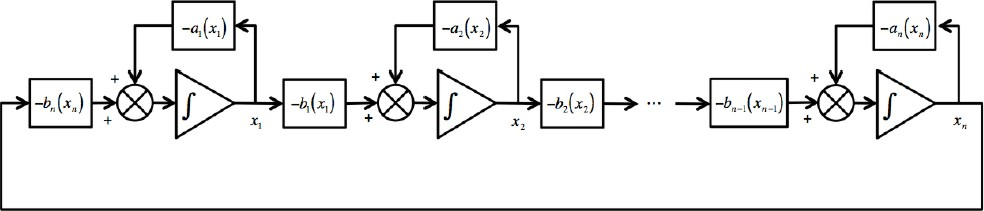Fig. 1 A particular class of cyclic feedback systems. In this case, the rate of change of each state in the chain results from the addition of a function which depends on the state itself plus a function which depends on the contiguous downstream state

where ai (·) and bi (·) are continuous functions (if these functions are real constants the corresponding system is obviously linear).

Remark 2: The cyclic feedback modular structure shown in (2) has been frequently considered to describe cyclic biochemical reactions, where the end product drives the first reaction, and with the functions ai (·) and bi (·) usually related to enzymatic processes. The cyclic feedback modular structure (2) is particularly suited for both the description and the design of biosynthetic oscillatory systems. Notice that for biochemical reactions each state takes positive values, i.e., xi ∈ ℜ+.

3.2 Synthesized Cyclic Feedback Transcriptional Networks

Biosynthetic oscillators based on transcriptional regulation are frequently built following the cyclic structure given by (2), with inhibition of gene expression as the basic regulatory mechanism (see for instance ). We must point out that common natural cell biochemical oscillators (e.g. circadian oscillators in animals and fungus), involve both transcriptional regulation and translational regulation (see for instance ).

Since the cell transcriptional machinery is easy to manipulate via standard recombinant DNA technologies, feedback-based transcriptional oscillators are preferred in cell biosynthetical constructs. We describe here the particular class of cyclic feedback systems that concerns our proposal. We focus our attention in cyclic feedback biosynthetic networks resulting from the chaining of transcriptional modules. Therefore, we first introduce the basic representation of a transcriptional module in input-output terms.

Consider x to be a gene, and let us assume that it codes the transcription factor1 [x], where [] stands for the concentration of the protein coded by . We can now recall the following (see for instance ):

Definition 1: In input–output terms, a transcriptional module maps an excitatory input signal consisted of the concentration of a transcription factor into an output–signal consisted also of the concentration of a expressed transcription factor. If we denote z and x the genes coding the input signal and the output signal, respectively, the dynamical behavior of the transcriptional module is described in lumped time-invariant terms by the set of coupled ordinary–differential-equations given by (see Figure 2):

where:

• — [rx] stands for the concentration of rx, the mRNA–molecules resulting from the transcription of gene x.

• f ([z]) denotes the excitatory signaling pro-cess promoted by the transcription factor [z].

• γrx is the constant translation rate from mRNA to the transcription factor coded by x.

• αx and αrx are the decay constant rates of [x] and [rx], respectively.Fig. 2 Schematic representation of a transcriptional module. The excitatory signal [z] binds to the promoter of gene x, which promotes changes in the expression of the corresponding transcription factor [x

Remark 3: The function f ([z]) captures the specificity of the regulatory role played by the transcription factor [z], and in general is nonlinear in nature. The regulatory function comes as a result of the interaction between [z] and the DNA–sequence which characterizes the promoter of the regulated gene x. Depending on the design, the regulatory process can involve inhibition or promotion through enzymatic dynamics.

We introduce now the class of cyclic feedback network of synthesized transcriptional modules that concerns us.

Definition 2: Cyclic feedback network of syn-thesized transcriptional modules] We define the class of n–th order cyclic feedback networks of synthesized transcriptional modules as the closed chains of transcriptional modules described as:

with:

corresponding to the i–th transcriptional module, and with:

The simple transcriptional modular structure given by (3) has been successfully applied in the construction of actual biosynthetic oscillators, with the transcription factors xi, i = 1, . . . , n, required to display sustained oscillations through the appropriate choice of the nonlinear regulatory functions fi, i = 1, . . . , n, and the right tuning of the parameters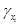,, and(i.e. the nonlinear regulatory functions as well as the parameters, including the order of the system, are chosen in order to guarantee that system (3) has a stable limit cycle). The celebrated repressilator (see for instance ) is a well–known example of this family of oscillatory modular transcriptional networks governed by negative feedback, see  and .

In the sequel we describe our inverse problem, which concerns the tuning of the mathematical model consisting of a cyclic feedback modular transcription network from information provided by a synthesized system derived from the model.

3.3 Finding the Parameter Values

In the previous subsection we presented the class of modularly structured cyclic feedback systems intended to guide the conception and construction of biosynthetic oscillators based on transcriptional regulation.

Problem 1. Consider a synthesized transcriptional network to be given and resulting from a mathematical description given by system (3) (i.e. we conceive the synthesized transcriptional network to be a designed cyclic feedback system). Assume that the synthesized transcriptional net-work displays sustained oscillatory behavior. From the knowledge of the state variables {[x1], [], [x2], [], . . ., [xn], []}, of the synthesized transcriptional network (i.e. the concentration of the transcription factors with their corresponding concentration of the mRNA transcripts), find a set of parameters {,,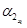}, for i = 1, 2, . . . , n, and the parameter values of the nonlinear transcription regulatory functions fi (·), for i = 1, 2, . . . , n, making a corresponding tuned mathematical model to closely describe the behavior of the synthesized transcriptional network.

Remark 4: We propose in the sequel a solution for Problem 1. We conceive our proposal as a proof–of–concept and because of that, and in order to simplify our exposition, we only consider here the case concerning the well-known synthetic bio-oscillator known as the repressilator.

The repressilator

In the case corresponding to the repressilator (see [8, 6]) n = 3 in (3), and the regulatory functions f1 (·), f2 (·) and f3 (·), correspond to inhibition actions. In the named symmetrical case it is assumed that the transcriptional regulatory functions (all of them with negative slope) satisfy:

with the real parameter α and m defining the inhibitory regulatory functions. Moreover, in order to simplify allare assumed to be equal to 1, and it is also assumed that the decay ratesandare equal to a real constant δ. Thus, the simplified symmetrical repressilator is described as follows:

Applying the Mallet–Paret and Smith theorems (see ), it is proved in  that this cyclic system has only one unstable equilibrium point and displays stable oscillatory behavior when the following inequality holds:

Let us now introduce our proposed tuning procedure.

4 Tuning Procedure

We consider here the symmetrical case for the repressilator (4)–(6), and we define the terms:

Our goal is to build a procedure to determine as precisely as possible the parameter vector P which tune the mathematical model (4)(6) in order to make it to display the oscillatory behavior observed from the known state vector X.

In order to manage the algebraic manipulations of the system equations (4)–(6) we define:

where

Evidently, from the system equations (4)–(6) we have that the parameter δ is algebraically identifiable (see for instance ) with respect to the output vector, Y , because:

That is, δ can be reconstructed with high precision. On the other hand, once the parameter δ is available, the time-variable regulatory signals fi(y) are algebraically observable, because:

It should be noticed that, if fi(y) is recovered, then α fulfills:

Therefore, the parameter m can be estimated by solving the following algebraic nonlinear equation:

where,and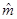are estimates of f(yi) and m, respectively.

Remark 5: From the previous analysis we conclude that the repressilator parameters can be recovered. Parameter δ can be then algebraically reconstructed with high precision, whereas parameters α and m can be estimated. It must be pointed out that our conclusion is related to the structural identifiability property introduced in , and is derived from theoretical developments first exposed in . Moreover, in the context of systems biology nonlinear identifiability (required for parameter reconstruction) has also been explored in .

We show now the procedure recovering the parameter vector P with respect to the known output vector Y .

4.1 Model-based On-line Parameters Estimation

Let us introduce the following assumptions (that we require in order to ensure the solvability of our systems inverse problem):

A1 The cyclic feedback system (4)–(6) is initialized to be strictly positive.

A2 The parameter vector P belongs to some neighborhood in the parameter space, such that, the system (4)–(6) exhibits sustained oscillatory behavior.

A3 The output vector Y is fully measurable.

We proceed now to expose our parameter recovering procedure.

On–line algebraic recovering of δ

This procedure consist of multiplying both sides of (11) by the time variable t, and then, integrating by parts the resulting expression. Thus, we have the expression:

By integrating once the left-hand side of the above equation we obtain the following linear expression in terms of the unknown parameter δ:

where

For sake of simplicity, we introduce the following notation:

Remark 6: Note that the system equation (16) is not well defined at time t = 0. But, for any time t after a small open time interval of the form (0, 𝜖) with 𝜖 > 0, the denominator of the equation (16) is strictly positive by assumptions A1 and A2. That is:

We focus our attention now on the computational procedure to recover the non-available regulatory signals fi, as well as the related parameters.

Reconstructing the time variable signals fi(y) and the unknown parameters α and m

As can be seen, the implementation of the relations in (12) needs the time derivative of the signals yi+3 to be recovered. To overcome this issue, we introduce the following simple high–gain observer (see ). Assuming that the signals yi are available, we can propose the following filter:

where ξ is a small positive parameter and k is a strictly positive parameter.

Proposition 1: Let yi(t) be a scalar continuous smooth function with its corresponding time derivatives satisfying || ≤ ni. Then, the high–gain observer recovers the time derivative of, with bounded error given by:

Then, the statesconverge to, when time elapses.

Proof: see Appendix A.

Consequently, the time variant regulatory signal fi can be reconstructed by:

whereare directly obtained from the filter (19). Since all the output signals, yi+3, present oscillatory behavior (which is one of our initial assumptions), this signals and their respective time derivatives are all bounded.

Now we are able to numerically solve the one variable nonlinear algebraic equation (14) and then be able to reconstruct the parameter m. To solve this equation we can apply the standard iterative Newton-Raphson method as follows:

where zy(m) is as defined in (14) and

Note that if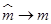, then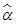can be computed using the formula in (13). That is:

Remark 7: Note that (21) requires to sample the outputs yi = xi, for i = {1, 2, 3}, since(see (14)). For this we define the indexed variables xik = xi(tk), where tk+1 − tk = T , with the sampling time T > 0 fixed. We computeas long as x1kx2kx3k.

We proceed now to illustrate our proposal with a numerical example.

5 Numerical Simulations

In this section, we test the effectivity of our proposed methodology via computer–based sim-ulations involving our chosen cyclic feedback system, the repressilator.

5.1 The Simulated Repressilator

We simulate the repressilator’s behavior choosing the parameter values: m = 1.5, δ = 1, and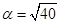(this selection guarantees sustained oscillatory behavior). As far as the initial conditions are arbitrary, we select them as:

5.2 Algebraic Reconstruction of the Parameter δ

The numerical implementation of our reconstructor is done by using the MatlabTM numerical computing environment. We use the Runge–Kutta method with an integration step of 0.5 × 103. The high-gain observer gains are fixed as ξ = 0.5×102 and k = 1. As already established, we need to recover the parameter δ first. In Figure 3, the reconstruction of parameter δ is shown.

As we can see, the parameter δ can be satis-factorily recovered regardless of the availability of measurements of,, and(in fact, notice that for the current illustrative example a set of measurements is at disposal for identification).

5.3 Estimation of the Non-available Regulatory Signals f1, f2 and f3

Now, since we effectively recover δ we are able to reconstruct the non–available regulatory signals f1, f2 and f3. Figure 4 shows the corresponding estimation errors for these three estimated signals. As we can see, the convergence time is very short because the high–gain observer gains were selected to be large enough.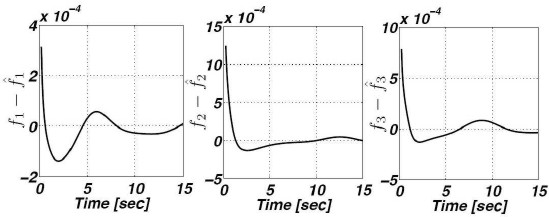Fig. 4 Estimation errors corresponding to the estimated signals f1, f2, and f3

5.4 Recovering Parameters m and α

Finally, having effectively estimated the regulatory signals b fi, the parameters m and α are effectively recovered, in that order, by solving the expression in (21) and (22) applying the Newton–Raphson method using samples of the regulatory signals b fi and xi every 0.5 seconds. As we can see, these parameters are in fact recovered with a very high accuracy (see Figure 5).

6 Conclusions

We proposed a methodology combining exact algebraic parameter reconstruction with nonlinear observed-based parameter estimation, intended to tune mathematical models guiding the design of synthetic biological circuits. We constrained our exposition to a class of cyclic modularly structured synthetic transcriptional networks which design is based on cyclic feedback systems described by deterministic lumped ordinary differential equations, and we illustrated our tuning procedure via computer–based simulations involving a low-dimensional cyclic feedback system corresponding to the celebrated synthetical transcriptional network called the repressilator. We focused our proposal to the minimization of the mismatch between the behavior of a synthesized biological circuit and the designed behavior coded by the mathematical model guiding the design process. The monitoring of the simulated synthesized biocircuit fed the information required to estimate the parameter values of the mathematical model.

We strongly believe that both exact algebraic parameter reconstruction and nonlinear observed-based parameter estimation are useful as a design tool for synthetic biology, since the solution of the systems inverse problem can be useful to close the gap between model–based designed biocircuits and implementations. As simple as it is, our methodology has been conceived as a proof-of-concept to illustrate how parameter tuning can be applied when considering the design of synthetic biocircuits when simple mathematical models are considered. It is quite obvious that realistic biocircuit design will involve the resolution of more complex systems inverse problems, particularly when phenomena like stochastically or retroactivity play a dominant role in the behavior of the involved systems.

To conclude, we must point out that the addition of identifiability properties to biocircuits may open the door to the inclusion of operational monitoring in biosynthetic networks, as a mechanism to promote functional reliability.

Acknowledgements

This research was supported by the Centro de Investigación en Computación of the Instituto Politecnico Nacional (CIC-IPN), and by the Secretaría de Investigación y Posgrado of the Instituto Politecnico Nacional (SIP-IPN), under Research Grant 20160268.

References

1. Anguelova, M (2004). Nonlinear observability and identifiability: General theory and a case study of a kinetic model for S. Cerevisiae. Chalmers University of Technology. [ Links ]

2. Arcak, M., & Sontag, E. D. (2006). Diagonal stability of a class of cyclic systems and its connection with the secant criterion. Automatica, Vol. 42, No. 9, pp. 1531–1537. [ Links ]

3. Carlson, R. H (2010). Biology is technology: the promise, peril, and new business of engineering life, volume 2010. Harvard University Press Cambridge, MA. [ Links ]

4. Dabroom, A. M., & Khalil, H. K. (1999). Discrete-time implementation of high-gain observers for numerical differentiation. International Journal of Control, Vol. 72, No. 17, pp. 1523–1537. [ Links ]

5. Del Vecchio, D., Ninfa, A. J., & Sontag, E. D. (2008). Modular cell biology: retroactivity and insulation. Molecular systems biology, Vol. 4, No. 1, pp. 161. [ Links ]

6. Del Vecchio, D., & Sontag, E. D. (2009). Synthetic biology: A systems engineering perspective. Control Theory and Systems Biology, pp. 101–124. [ Links ]

7. Elowitz, M., & Lim, W. A. (2010). Build life to understand it. Nature, Vol. 468, No. 7326, pp. 889–890. [ Links ]

8. Elowitz, M. B., & Leibler, S. (2000). A synthetic oscillatory network of transcriptional regulators. Nature, Vol. 403, No. 6767, pp. 335–338. [ Links ]

9. Endy, D (2005). Foundations for engineering biology. Nature, Vol. 438, No. 7067, pp. 449–453. [ Links ]

10. Fliess, M., & Sira-Ramırez, H. (2004). Recon-structeurs d’état. Comptes Rendus Mathematique, Vol. 338, No. 1, pp. 91–96. [ Links ]

11. Gedeon, T (1998). Cyclic feedback systems, volume 637. American Mathematical Soc. [ Links ]

12. Hermann, R., & Krener, A. J. (1977). Nonlinear controllability and observability. IEEE Transactions on automatic control, Vol. 22, No. 5, pp. 728–740. [ Links ]

13. Kim, J., & Winfree, E. (2011). Synthetic in vitro transcriptional oscillators. Molecular systems biology, Vol. 7, No. 1, pp. 465. [ Links ]

14. Lakin-Thomas, P. L (2006). Transcriptional feed-back oscillators: maybe, maybe not... Journal of biological rhythms, Vol. 21, No. 2, pp. 83–92. [ Links ]

15. Mallet-Paret, J., & Smith, H. L. (1990). The poincaré-bendixson theorem for monotone cyclic feedback systems. Journal of Dynamics and Differential Equations, Vol. 2, No. 4, pp. 367–421. [ Links ]

16. Martínez-García, J. C., Aguilar-Ibañez, C. F., Cabello-Sánchez, U., & Soria-López, A. (2012). Tuning of mathematical models describing synthetic cyclic feedback biocircuits: combining exact algebraic parameter reconstruction and nonlinear parameter estimation. 20th International Symposium on Mathematical Theory of Networks and Systems (MTNS’2012), Melbourne, Australia. [ Links ]

17. Myers, C. J (2009). Engineering genetic circuits. CRC Press. [ Links ]

18. Shetty, R. P (2008). Applying engineering princi-ples to the design and construction of transcriptional devices. Ph.D. thesis, Massachusetts Institute of Technology. [ Links ]

19. Smolke, C. D., & Silver, P. A. (2011). Informing biological design by integration of systems and synthetic biology. Cell, Vol. 144, No. 6, pp. 855–859. [ Links ]

1A transcription factor is a sequence-specific DNA-binding protein.

2Corresponding author is Carlos Aguilar-Ibanez.

Appendix A: Proof of Proposition 1

We define the vector error as:

where. Therefore, the dynamic errors can be expressed after using (19), as follows:

with

As can be seen, A𝜖 is a Hurwitz matrix, for all k > 0 and 𝜖 > 0. Thus, error ξi satisfies:

Since A𝜖 is exponentially stable and the signalis bounded, then the following inequality holds

where the constant β is as previously defined. This concludes the proof.

Received: May 25, 2016; Accepted: October 12, 2016This is an open-access article distributed under the terms of the Creative Commons Attribution License